Ex 5.1

Chapter 5 Class 11 Complex Numbers
Serial order wise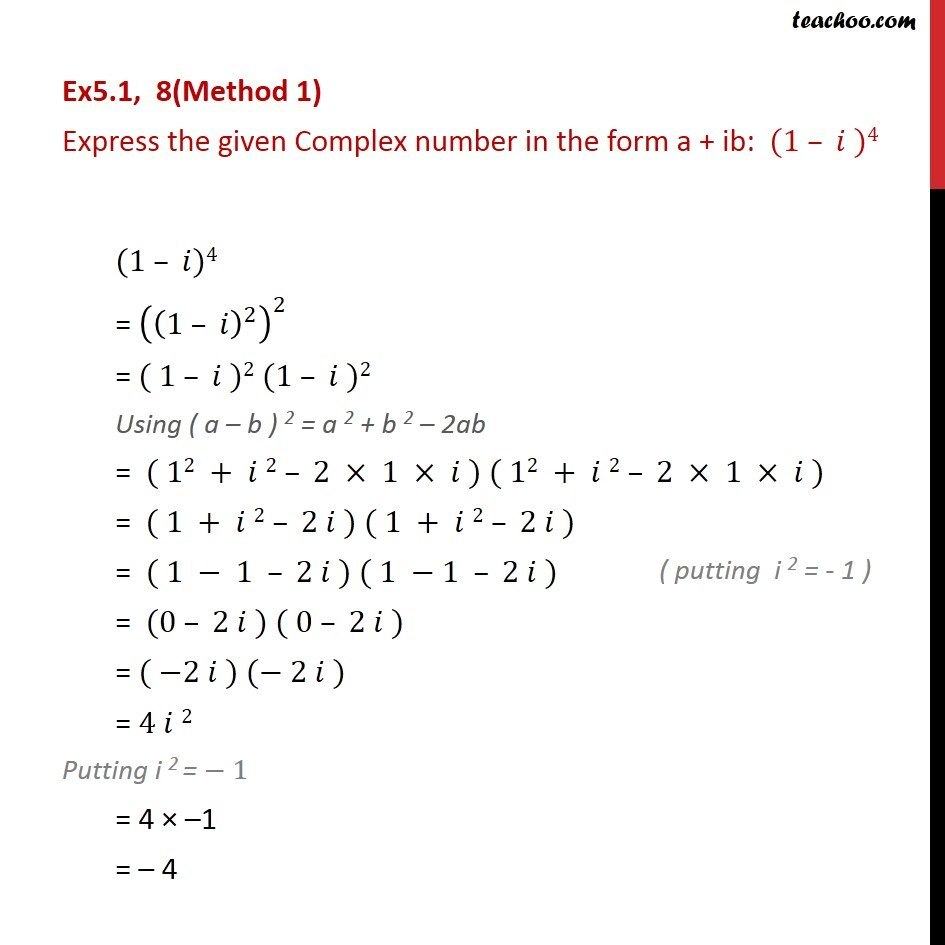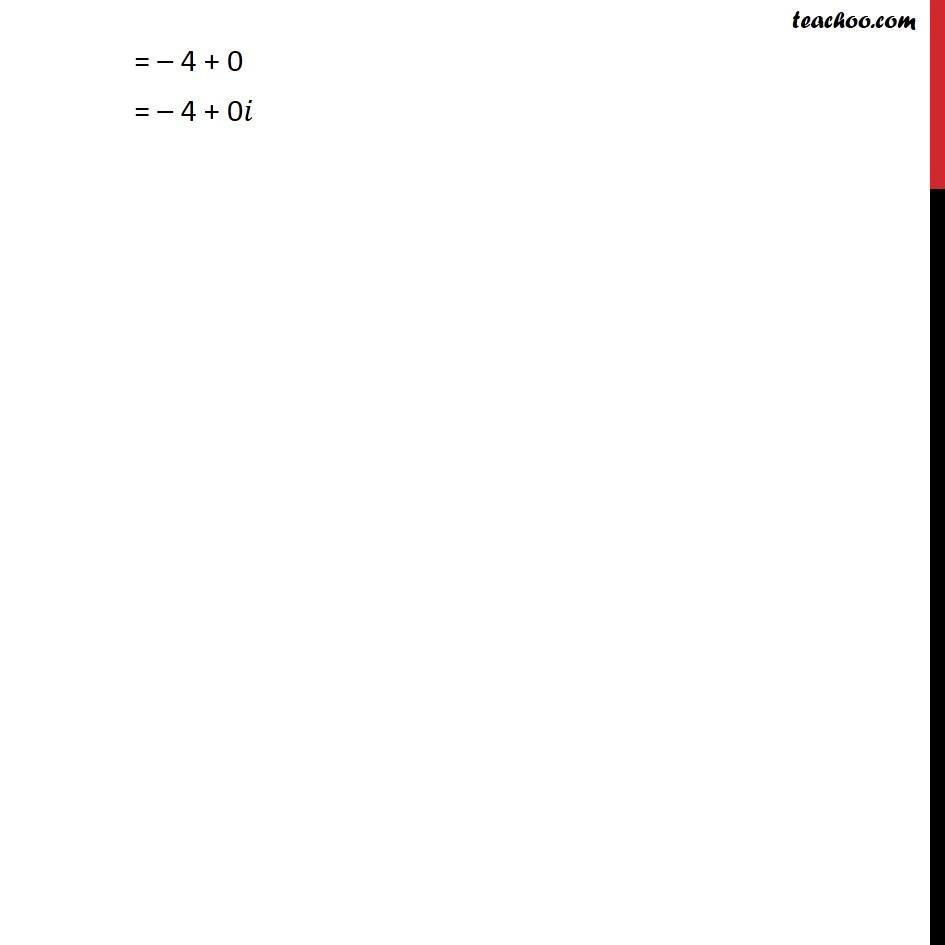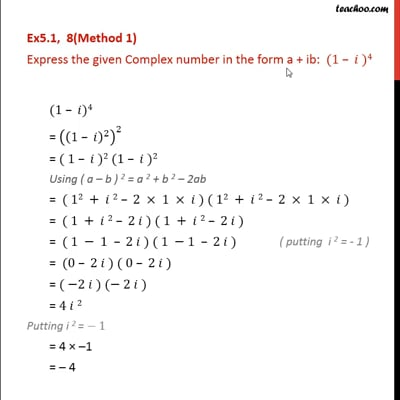This video is only available for Teachoo black users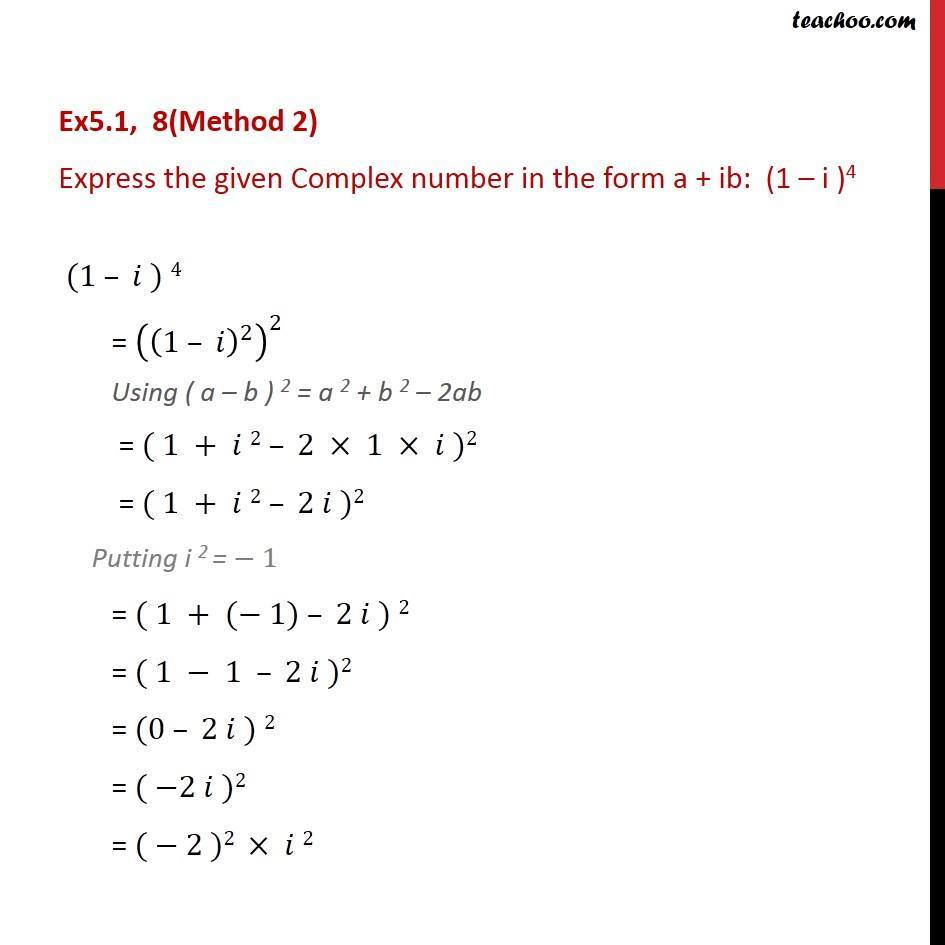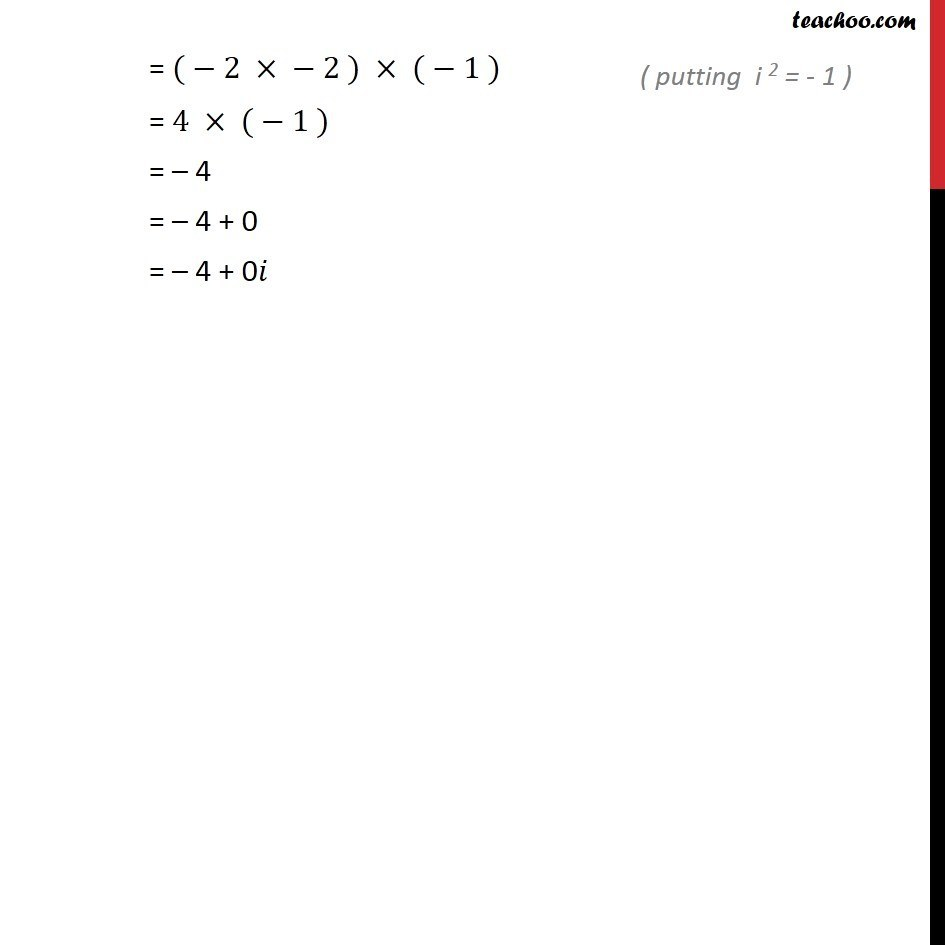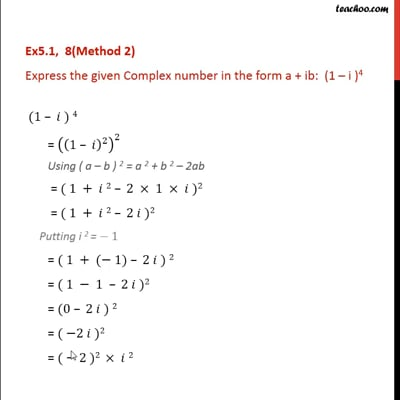This video is only available for Teachoo black users

Solve all your doubts with Teachoo Black (new monthly pack available now!)

### Transcript

Ex5.1, 8(Method 1) Express the given Complex number in the form a + ib: (1 )4 (1 )4 = ((1 )^2 )^2 = ( 1 )2 (1 )2 Using ( a b ) 2 = a 2 + b 2 2ab = ( 12 + 2 2 1 ) ( 12 + 2 2 1 ) = ( 1 + 2 2 ) ( 1 + 2 2 ) = ( 1 1 2 ) ( 1 1 2 ) = (0 2 ) ( 0 2 ) = ( 2 ) ( 2 ) = 4 2 Putting i 2 = 1 = 4 1 = 4 = 4 + 0 = 4 + 0 Ex5.1, 8(Method 2) Express the given Complex number in the form a + ib: (1 i )4 (1 ) 4 = ((1 )^2 )^2 Using ( a b ) 2 = a 2 + b 2 2ab = ( 1 + 2 2 1 )2 = ( 1 + 2 2 )2 Putting i 2 = 1 "= "( 1 + ( 1) 2 ) 2 "=" ( 1 1 2 )2 "=" (0 2 ) 2 "=" ( 2 )2 "=" ( 2 )2 2 = ( 2 2 ) ( 1 ) = 4 ( 1 ) = 4 = 4 + 0 = 4 + 0